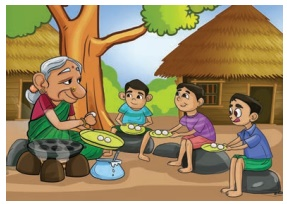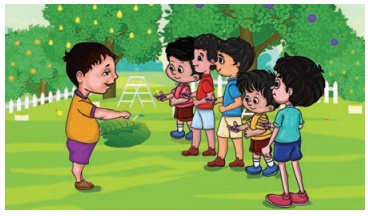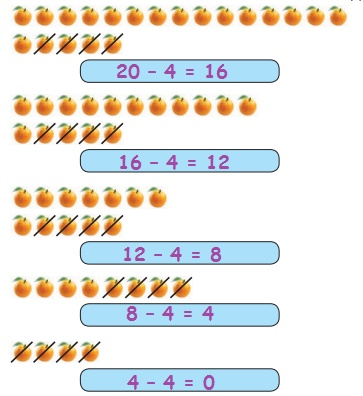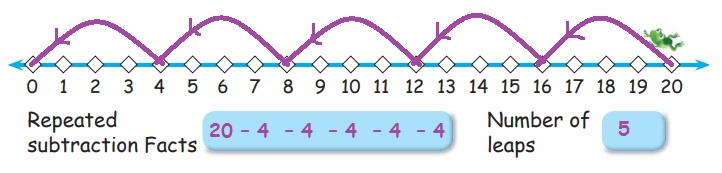Home | | Maths 2nd Std | Repeated Subtraction

# Repeated Subtraction

Ezhilan, Kumaran and Iniyan went to grandma chinnathai’s house for holiday. She prepared tasty paniyarams for them. Observe the way she shared the paniyarams with them. How many paniyarams does each child get?

Repeated Subtraction

Travel Through

Ezhilan, Kumaran and Iniyan went to grandma chinnathai’s house for holiday. She prepared tasty paniyarams for them. Observe the way she shared the paniyarams with them. How many paniyarams does each child get?Kumaran : Wow! Grandma! Paniyarams, I love them.

Ezhilan : I want more.

Iniyan : No ! I want more.

Grandma : Don‛t worry children. I will give you equally.Grandma : I have 9 paniyarams. I will give you one by one.

Ezhilan : All of us got 1 and 3 paniyarams are over.

Kumaran : You have 6 Paniyarams grandma.

Iniyan : We can write the same as 9 – 3 = 6.Grandma : This is the second round.

Iniyan : You can again give us one paniyaram each, granny.

Kumaran : Now, You have 3 paniyarams left grandma.

Ezhilan : This can be written as 6 – 3=3.Grandma : Yes! Then I guess I can give another round. This is the third round.

Ezhilan : Wow ! The third one grandma.

Kumaran : We all have three paniyarams each and your plate is empty grandma.

Iniyan : So, it is 3 – 3 = 0.

So, 9 – 3 = 6; 6 – 3 = 3; 3 – 3= 0.

From 9 we can take away 3 paniyarams 3 times.

Learn

One day, Ravi saw a tree full of jamun fruits. The gardener gave him more fruits.He counted them. There were 20 fruits.

Ravi has 20 jamun fruits. He wants to give them to 5 of his friends. He distributed them one by one.First, He gave one jamun fruit to each of them which can be expressed as 20 – 5=15.Ravi is left with 15 jamun fruits.

He gives one more jamun fruit to each of them in the second time

This can be written as 15 – 5=10.Now, Ravi is left with 10 jamun fruits. He again gives 1 jamun fruit to each of his 5 friends. We write this as 10 – 5=5.Now, Ravi is left with 5 jamun fruits.

He again gives 1 jamun fruit to each of his 5 friends. We write this as 5 – 5=0. Finally, Ravi’s bag is empty.

He gives 5 fruits four times.

He can distribute 20 fruits to 5 of his friends 4 times.

Practice

1. If grandma chinnathai had 12 paniyarams, how many paniyarams will each one of her 3 grandsons get?

To begin with, we start with 12 paniyarams and distribute them to 3 grandsons and so 12 – 3= 9. Complete the remaining repeated subtraction facts in the table given below.12 –  3 = 9

9 – 3 = 6

6 – 3 = 3

3 – 3 = 0

Each grandson gets  4 paniyarams.

2. If grandma had 20 paniyarams, and there are 4 children, how many paniyarams will each child get?

Complete the subtraction facts in the table given below..20 – 4 = 16

16 – 4 = 12

12 – 4 = 8

8 – 4 = 4

4 – 4 = 0

Each child gets  5 paniyarams.

Pleasure Time

Write the subtraction facts for following.

i. Subtract 2 repeatedly from 1010 – 2 = 8

8 – 2 = 6

6 – 2 = 4

4 – 2 = 2

2 – 2 = 0

Number of times ‘2’ is subtracted to get 0 5 times

ii. Subtract 4 repeatedly from 2020 – 4 = 16

16 – 4 = 12

12 – 4 = 8

8 – 4 = 4

4 – 4 = 0

Number of times ‘4’ is subtracted to get 0 5 times

Learn

Let us do repeated subtraction using number line.The frog can leap 3 units in one attempt. If the frog wants to return to its original position from 15 then it can be shown using number line as given above.Start from 15 and leap in three’s upto 0.

Frog will reach 0 in 5 leap of 3 units each.

It has jumped 5 times starting from 15 to reach 0. That is 15 – 3 =12;

12 – 3 = 9; 9 – 3= 6; 6 – 3 = 3; 3 – 3 = 0, which gives 15 – 3 – 3 – 3 – 3 – 3 = 0

Practice

Draw the leaping position of the frog on the line to return to 0.

i) 2 units distanceRepeate subtraction Facts : 20 – 2 – 2 – 2 – 2 – 2 – 2 – 2 – 2 – 2 – 2

20 – 2 = 18

18 – 2 = 16

16 – 2 = 14

14 – 2 = 12

12 – 2 = 10

10 – 2 = 8

8 – 2 = 6

6 – 2 = 4

4 – 2 = 2

2 – 2 = 0

Number of leaps 10

ii) 5 units distanceRepeated subtraction  Facts : 20 – 5 – 5 – 5 – 5

20 – 5 = 15

15 – 5 = 10

10 – 5 = 5

5 – 5 = 0

Number of Leaps 4

iii) 4 units distanceRepeated subtraction Facts: 20 – 4  – 4  – 4  – 4  – 4

20 – 4 = 16

16 – 4 = 12

12 – 4 = 8

8 – 4 = 4

4 – 4 = 0

Number of Leaps 5

Mental Maths

1. Vanitha had 50 mangoes and she made packets of five mangoes each and sold them. How many packets does she make?50 – 5 = 45 - 1st Packet

45 – 5 = 40 - 2nd Packet

40 – 5 = 35 - 3rd Packet

35 – 5 = 30 - 4th Packet

30 – 5 = 25 - 5th Packet

25 – 5 = 20 - 6th Packet

20 – 5 = 15 - 7th Packet

15 – 5 = 10 - 8th Packet

10 – 5 = 5 - 9th Packet

5 – 5 = 0 - 10th Packet

Ans : 10 packets

2. There are 30 notebooks in a box. If 6 notebooks are to be given to each student, how many students will get the note books?30 – 6 = 24 - 1st student

24 – 6 = 18 - 2nd student

18 – 6 = 12 - 3rd student

12 – 6 = 6 - 4th student

30 – 6 = 0 - 5th student

Ans : 5 students

3. 20 members planned to cross the river by boat. Each boat can carry 4 members. How many time the boat needs to take them?20 – 4 = 16 - 1st boat

16 – 4 = 12 - 2nd boat

12 – 4 = 8 - 4th boat

8 – 4 = 4 - 3rd boat

4 – 4 = 0 - 5th boat

Ans : 5 time

4. Mani has 15 rupees with him. If he wants to buy newspaper by spending 3 rupees a day, for how many days can he buy the news papers?15 – 3 = 12 - 1st day

12 – 3 = 9 - 2nd day

9 – 3 = 6 - 3rd day

6 – 3 = 3 - 4th day

3 – 3 = 0 - 5th day

Ans : 5 days

5. Kavya’s uncle gave her 20 date fruits and asked her to eat 2 fruits a day. How many days can she eat the fruits?20 – 2 = 18 - 1st day

18 – 2 = 16 - 2nd day

16 – 2 = 14 - 3rd day

14 – 2 = 12 - 4th day

12 – 2 = 10 - 5th day

10 – 2 = 8 - 6th day

8 – 2 = 6 - 7th day

6 – 2 = 4 - 8th day

4 – 2 = 2 - 9th day

2 – 2 = 0 - 10th day

Ans : 10 days

Tags : Numbers | Term 3 Chapter 1 | 2nd Maths , 2nd Maths : Term 3 Unit 1 : Numbers
Study Material, Lecturing Notes, Assignment, Reference, Wiki description explanation, brief detail
2nd Maths : Term 3 Unit 1 : Numbers : Repeated Subtraction | Numbers | Term 3 Chapter 1 | 2nd Maths